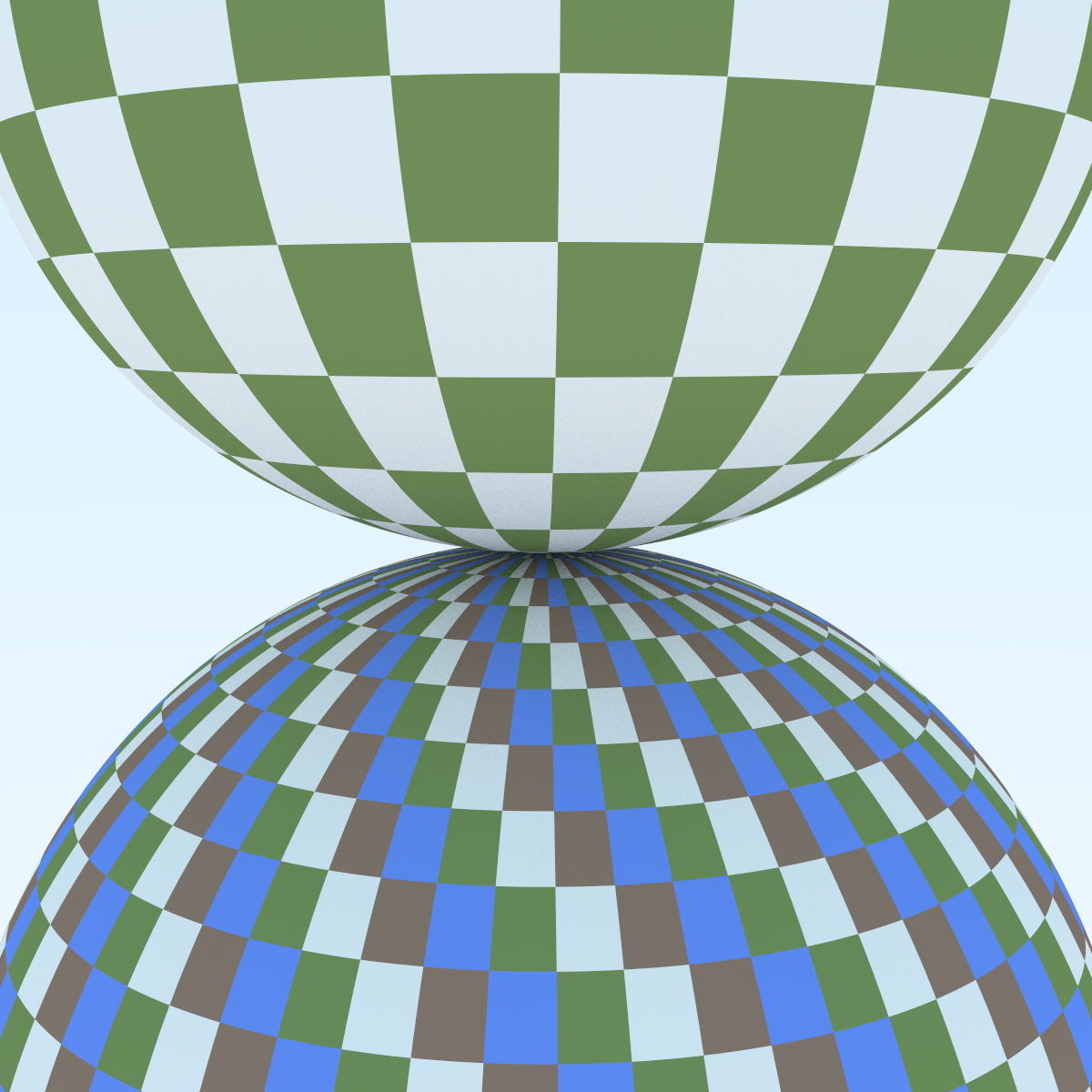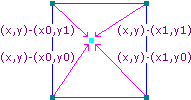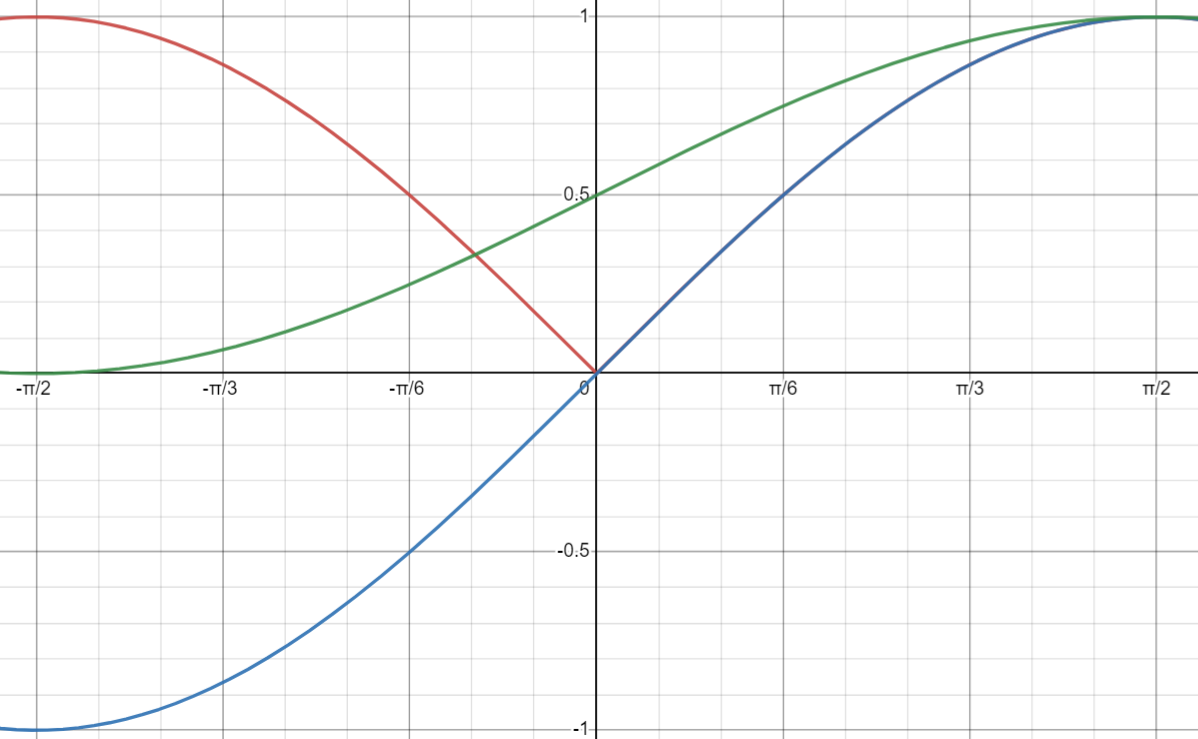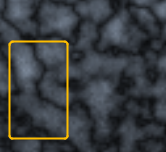# bvh_node

bvh_node::bvh_node(
std::vector<shared_ptr<hittable>>& src_objects,
size_t start, size_t end, double time0, double time1
)

std::sort(objects.begin() + start, objects.begin() + end, comparator);

std::sort() 会排序的其实是 [) 这样的一个区间（我之前居然没注意到这个）。所以这里的 objects.begin() + end 其实没有包括 end

# 球面纹理坐标

class sphere : public hittable {
...
private:
static void get_sphere_uv(const point3& p, double& u, double& v) {
// p: a given point on the sphere of radius one, centered at the origin.
// u: returned value [0,1] of angle around the Y axis from X=-1.
// v: returned value [0,1] of angle from Y=-1 to Y=+1.
//     <1 0 0> yields <0.50 0.50>       <-1  0  0> yields <0.00 0.50>
//     <0 1 0> yields <0.50 1.00>       < 0 -1  0> yields <0.50 0.00>
//     <0 0 1> yields <0.25 0.50>       < 0  0 -1> yields <0.75 0.50>

auto theta = acos(-p.y());
auto phi = atan2(-p.z(), p.x()) + pi;

u = phi / (2*pi);
v = theta / pi;
}
};

## 棋盘格纹理

### 书中的实现

virtual color value(double u, double v, const point3& p) const override {
auto sines = sin(10*p.x())*sin(10*p.y())*sin(10*p.z());
if (sines < 0)
return odd->value(u, v, p);
else
return even->value(u, v, p);
}$y=\operatorname{mod}\left(x,100\right)-50$

### 仅在表面的棋盘格

class surface_checker : public texture {
public:
using text_array = std::vector<std::shared_ptr<texture>>;
surface_checker() = default;
surface_checker(const text_array& _texts,
const std::pair<f8, f8> _siz = {514, 114})
: texts(_texts), polar_azim_siz(_siz) {}

virtual color value(f8 polar, f8 azim, const pt3& p) const override {
int x_idx = (i8)(azim * polar_azim_siz.first);
int y_idx = (i8)(polar * polar_azim_siz.second / 2.0);
// 极角只跨半球，所以想要整个球的垂直方向有 polar_azim_siz.second 这么多的格子，要先除以二
return texts[(x_idx + y_idx) % texts.size()]->value(polar, azim, p);
}

text_array texts;
std::pair<f8, f8> polar_azim_siz;  // 垂直方向和水平方向有多少格
};scene surf_check_sc() {
hittable_list world;

auto checker1 = make_shared<surface_checker>(
surface_checker::text_array{
make_shared<fixed_color>(color(0.2, 0.3, 0.1)),
make_shared<fixed_color>(color(0.9, 0.9, 0.9)),
make_shared<fixed_color>(color(0.3, 0.2, 0.15)),
make_shared<fixed_color>(color(0.15, 0.3, 0.9))},
std::pair<f8, f8>{60, 60});

auto checker2 = make_shared<surface_checker>(
surface_checker::text_array{
make_shared<fixed_color>(color(0.2, 0.3, 0.1)),
make_shared<fixed_color>(color(0.9, 0.9, 0.9)),
},
std::pair<f8, f8>{30, 30});

make_shared<lambertian>(checker1)));
make_shared<lambertian>(checker2)));

f8 asp_ratio = 1.0;
vec3 lookfrom = pt3(13, 2, 3) * 2;
vec3 lookat = pt3(0, 0, 0);
f8 vfov = 40.0;
auto dist_to_focus = 10.0;
auto aperture = 0;
vec3 vup(0, 1, 0);
auto cam_ptr = make_shared<camera>(lookfrom, lookat, vup, vfov, asp_ratio,
aperture, dist_to_focus, aperture, 1.0);

return scene(make_shared<bvh_node>(world), blue_sky_back_ptr, cam_ptr);
}

# 柏林噪声import numpy as np
import matplotlib.pyplot as plt
from math import *

XLEN = 25 # 产生多少个整数点
YLEN = 25
DIFF = 0.05

ptsx = np.arange(0, XLEN, DIFF)
ptsy = np.arange(0, YLEN, DIFF)
xs, ys = np.meshgrid(ptsx, ptsy)
z_orig = np.random.random((XLEN + 1, YLEN + 1))
z_interped = np.zeros((round((XLEN) / DIFF), round(YLEN / DIFF)))

def lerp(a, b, t):
return a + t * (b - a)

def lerp2(ld, rd, lu, ru, tx, ty): # 二维线性插值
# left down, right down, left up, right up
upmid = lerp(lu, ru, tx)
dnmid = lerp(ld, rd, tx)
return lerp(dnmid, upmid, ty)

for i in range(XLEN):
for si in range(round(1 / DIFF)):  # step i
for j in range(YLEN):
for sj in range(round(1 / DIFF)):
z_interped[i * round(1 / DIFF) + si][j * round(1 / DIFF) + sj] = lerp2(
z_orig[i][j], z_orig[i + 1][j], z_orig[i][j + 1],  z_orig[i + 1][j + 1], DIFF * si, DIFF * sj)

plt.imshow(z_interped, cmap=plt.cm.gray)
plt.savefig("./2d.png", dpi = 150, format = 'png')
plt.show()$\vec{v_i} = \vec{d_i} \cdot \vec{g_i}$• 红框中大部分是黄色的，因为这部分点的距离向量和关键点的随机向量有相似的方向。
• 黄框中大部分是蓝色的，因为其左下角关键点随机向量的尾部指向了这片区域，也就是这片区域的距离向量和关键点随机向量相反。
• 绿框中的大部分是黄色的，虽然这片区域是左边关键点随机向量的反方向，但因为线性插值的存在，并且这片区域离右边的随机向量更近，其受到右边随机向量的影响更大。

## 湍流（turbulence）

double turb(const point3& p, int depth=7) const {
auto accum = 0.0;
auto temp_p = p;
auto weight = 1.0;

for (int i = 0; i < depth; i++) {
accum += weight*noise(temp_p);
weight *= 0.5;
temp_p *= 2;
}

return fabs(accum);
}### 一些疑问

Noise()
In order to get the most out of the PSE and the solid texture approach we have provided some primitive stochastic functions with which to bootstrap visual complexity. We now introduce the most fundamental of these. Noise() is a scalar valued function which takes a three dimensional vector as its argument. It has the following properties :

• Statistical invariance under rotation (no matter how we rotate its domain, it has the same statistical character)
• A narrow bandpass limit in frequency (its has no visible features larger or smaller than within a certain narrow size range)

Appendix. Turbulence
A suitable procedure for the simulation of turbulence using the Noise() signal is :

function turbulence(p)
t = 0
scale = 1
while (scale > pixelsize)
t += abs(Noise(p / scale) * scale)
scale /= 2
return t

By evaluating Noise() at visible surface points of simulated objects we may create a simple “random” surface texture (figure Spotted.Donut) :
color = white * Noise(point)

# 实例变换

## 旋转矩阵

### 公式推导

$x^\prime = \cos(\theta) - \sin(\theta) \cdot y \\ y^\prime = \sin(\theta) + \cos(\theta) \cdot y$

$x = r\cos\phi \\ y = r\sin\phi$

$x^\prime = r\cos(\phi + \theta) \\ y^\prime = r\sin(\phi + \theta)$

$\cos(\phi + \theta) = \cos\phi\cos\theta - \sin\phi\sin\theta \\ \sin(\phi + \theta) = \sin\phi\cos\theta + \cos\phi\sin\theta$

\begin{align*} x^\prime &= r(\cos\phi\cos\theta - \sin\phi\sin\theta) \\ x^\prime &= (r\cos\phi)\cos\theta - (r\sin\phi)\sin\theta \\ x^\prime &= x\cos\theta - y\sin\theta \end{align*}

\begin{align*} y^\prime &= r(\sin\phi\cos\theta + \cos\phi\sin\theta) \\ y^\prime &= r(\sin\phi)\cos\theta + r(\cos\phi)\sin\theta \\ y^\prime &= y\cos\theta + x\sin\theta \\ &= x\sin\theta + y\cos\theta \end{align*}

### 一些解释

$x$ 轴的旋转基本和这个没区别，但是绕 $y$ 轴的比较令人疑惑了。

           y+
|
|
|
x- ------- z --------- x+
|
|
|
y-

           y+
|
|
|
z+ ------- x --------- z-
|
|
|
y-

\begin{align*} y^\prime &= \cos \theta \cdot y - \sin\theta \cdot z \\ &= \cos(90) \cdot 1 - \sin(90) \cdot 0 \\ &= 0 - 0 = 0 \end{align*}

\begin{align*} z^\prime &= \sin \theta \cdot x + \cos \theta \cdot z \\ &= \sin(90) \cdot 1 + \cos(90) \cdot z \\ &= 1 + 0 = 1 \end{align*}

           z-
|
|
|
x- ------- y --------- x+
|
|
|
z+

\begin{align*} z^\prime &= \sin \theta \cdot x + \cos \theta \cdot z \\ &= \cos(90) \cdot 0 - \sin(90) \cdot -1 \\ &= 0 - (1 \cdot -1) = 1 \end{align*}